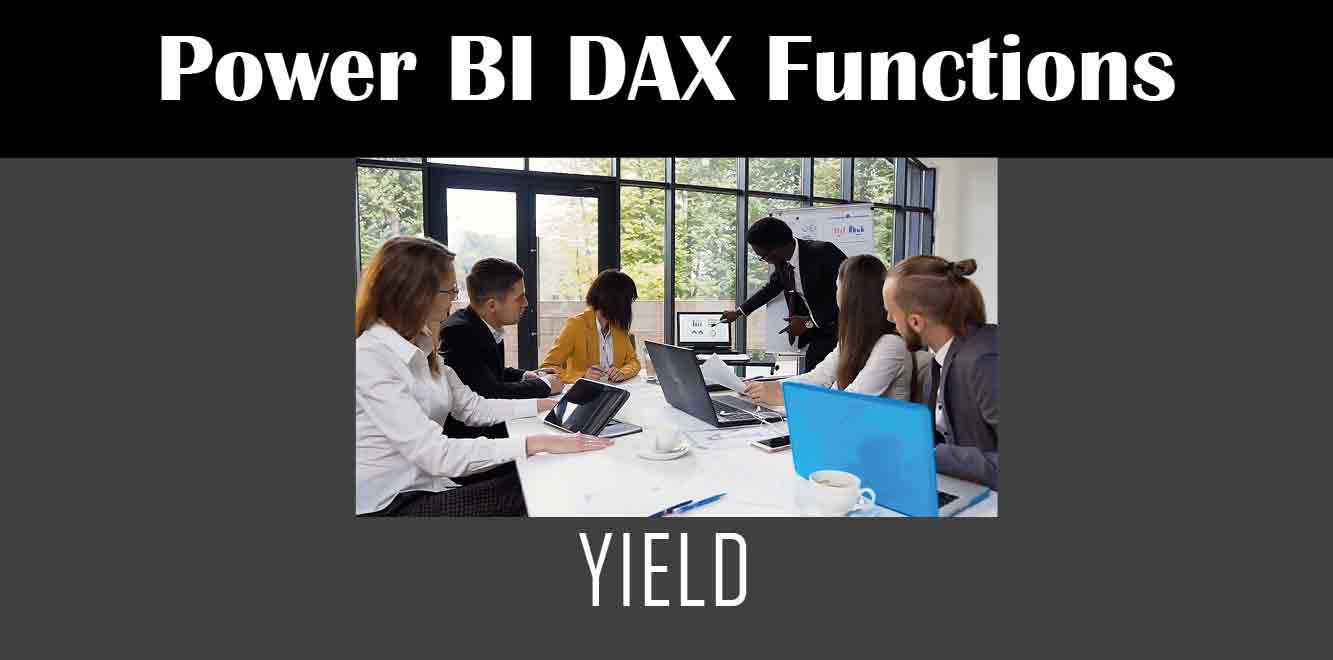# YIELD

## Y## Understanding the YIELD Function

The YIELD function in DAX calculates the yield of a financial instrument such as a bond. It is used to calculate the annual percentage rate of return on an investment. It takes four arguments: settlement, maturity, rate, and price. Settlement is the date on which the investment is made, maturity is the date on which the investment will mature, rate is the interest rate, and price is the price of the investment.

The formula for the YIELD function is:

YIELD(settlement, maturity, rate, price)

``` The result of the YIELD function is a decimal number, which represents the annual percentage rate of return on the investment. Using the YIELD Function in Power BI To use the YIELD function in Power BI, follow these steps: 1. Open Power BI Desktop and create a new report. 2. Open the formula bar by clicking on the fx button. 3. Type the YIELD formula in the formula bar, along with the required arguments. 4. Press Enter to calculate the yield. For example, if you want to calculate the yield on an investment that settles on 1/1/2021, matures on 1/1/2026, has an interest rate of 5%, and a price of \$1000, you would use the following formula: ```

YIELD(“1/1/2021”, “1/1/2026”, 0.05, 1000)

``` After pressing Enter, the result will be displayed in the formula bar. The YIELD function in DAX is a powerful tool for calculating the yield of financial instruments in Power BI. It is easy to use and can provide valuable insights into investment returns. By following the steps outlined in this article, you can use the YIELD function to calculate the yield on your investments in Power BI. Power BI DAX Training Courses by G Com Solutions (0800 998 9248)Power BI DAX Intensive Training Course £1,050.00 – £26,550.00 Select optionsContinue Loading DonePower BI DAX Introduction £395.00 – £9,750.00 Select optionsContinue Loading DonePower BI DAX Intermediate £395.00 – £9,750.00 Select optionsContinue Loading DonePower BI DAX Advanced £395.00 – £9,750.00 Select optionsContinue Loading Done Upcoming Courses Contact Us Subject Your Name (required) Company/Organisation Email (required) Telephone Training Course(s) Power BI Intensive TrainingPower BI introduction Power BI IntermediatePower BI AdvancedDAXPower Query MPower BI CertificationPower BI AdministrationPower PlatformPower AutomatePower AppsOTHER Your Message Upload Example Document(s) (Zip multiple files) ```
``` ```
``` ```
``` ```
``` ```
``` ```